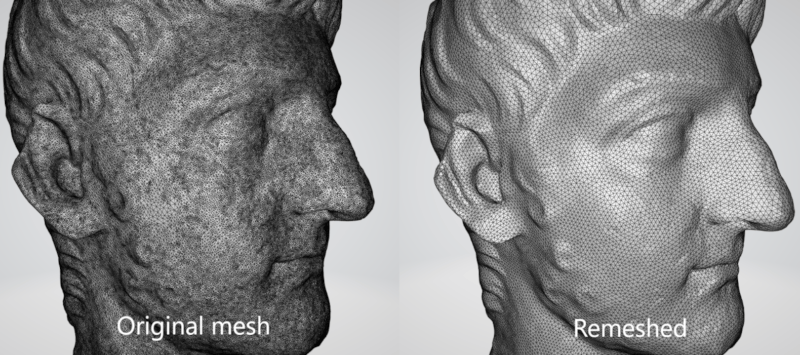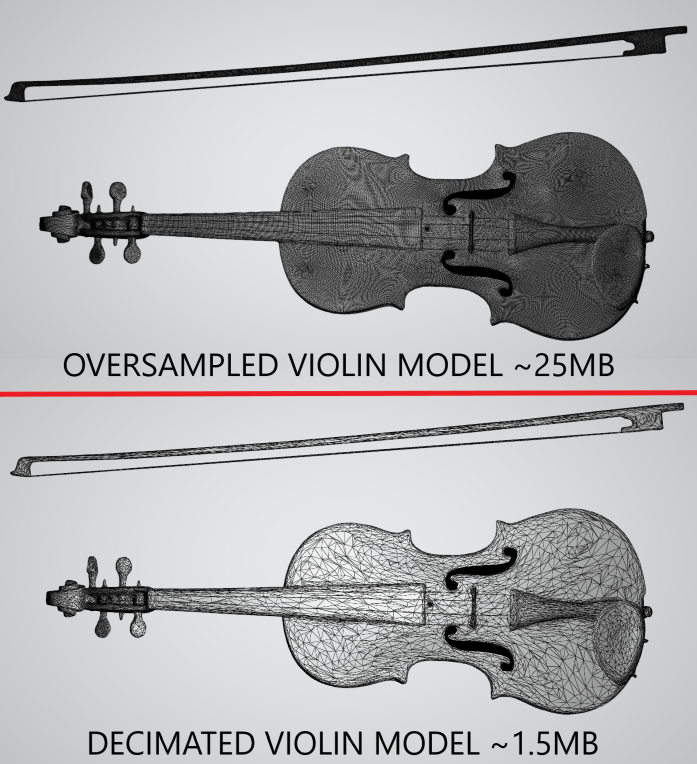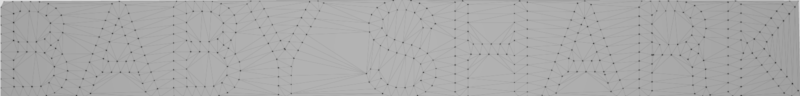## baby_shark

Geometry processing library

### 5 releases

 new 0.1.4 May 28, 2023 May 20, 2023 May 14, 2023 Mar 12, 2023 Mar 12, 2023

#282 in Math

325KB
7K SLoC

# Geometry processing library in pure Rust

## Features

• Corner table implementation for efficient mesh traversal
• Remeshing
• Mesh simplification (decimation)
• 2D triangulation
• Spatial index
• Bounding volume hierarchy of axis aligned bounding boxes
• Infinite grid

## Reading/writing mesh from/to STL file

You can read/write STL files using `StlReader` and `StlWriter` structs. Ony binary STLs are supported.

### Example

``````use std::path::Path;

use baby_shark::{
mesh::corner_table::prelude::CornerTableF
};

fn main() {

let writer = StlWriter::new();
writer.write_stl_to_file(&mesh, Path::new("./write.stl"))
.expect("Save mesh to STL file");
}
``````

## Isotropic remeshing

This algorithm incrementally performs simple operations such as edge splits, edge collapses, edge flips, and Laplacian smoothing. All the vertices of the remeshed patch are reprojected to the original surface to keep a good approximation of the input. Any of those operations can be turned off using appropriate method (`with_<operation>(false)`).### Example

``````let remesher = IncrementalRemesher::new()
.with_iterations_count(10)
.with_split_edges(true)
.with_collapse_edges(true)
.with_flip_edges(true)
.with_shift_vertices(true)
.with_project_vertices(true);
remesher.remesh(&mut mesh, 0.002f32);
``````

## Mesh simplification (decimation)

This library implements incremental edge decimation algorithm. On each iteration edge with lowest collapse cost is collapsed. Several stop condition are supported:

• Max error - algorithm stops when collapse lowest cost is bigger than given value
• Min faces count - algorithm stops when faces count drops below given value### Example

`````` let mut decimator = EdgeDecimator::new()
.max_error(Some(0.0005))
.min_faces_count(Some(10000));
decimator.decimate(&mut mesh);
``````

## 2D triangulation

`Triangulation2` struct implements fast 2D delaunay triangulation of points set.### Example

``````let mut triangulation = Triangulation2::new();
triangulation.triangulate(&vec![
Point2::new(1.0, 2.0),
Point2::new(5.0, 1.0),
Point2::new(8.0, 6.0),
Point2::new(2.0, 8.0)
]);
``````

~5MB
~106K SLoC